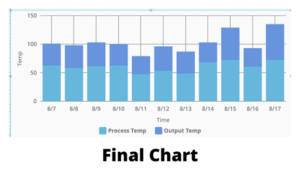Our goal is to plot both process temp and outside temp represented as bars, one on top of the other, aka a stacked bar chart. A successful implementation will look like figure 1 which displays process temp & output temp as stacked bars.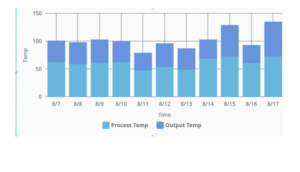Figure 1

Here are some detailed steps for creating a stacked bar chart in perspective:

### Step: #1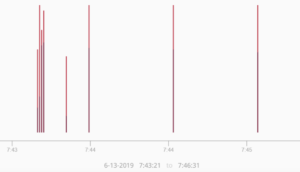Figure 2

The default timeseries bar chart does look like a stacked bar chart however, it has limitations.

Figure 2 shows one of the biggest limitations of the time series bar chart. The width of the bar chart is calculated automatically based on the amount of data already on the x- axis, so if you have sparse data with a wide time range then this bar chart will end up as thin vertical lines.

### Step: #2

Next we need to check how the database is configured.

The database needs to be configured with different categories that have columns.

Figure 3 shows an example of database data. If there are 2 different categories of alarms, then the database should have 2 separate columns matching the total number of alarms.

For example: Process Temperature & Output Temperature.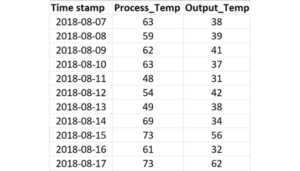Figure 3

### Step: #3

To keep things simple, let’s start with the dataset that comes with the default XY chart when you drag it in from the perspective components window. Figure 4 shows the default XY chart.Figure 4

### Step: #4

Next let’s change the Y-axis. Go to series 0 and 1, configure them to be plotted on the same Y-axis which is highlighted in figure 5.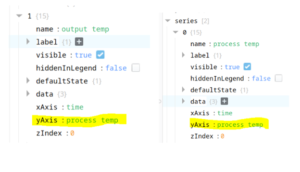Figure 5

### Step: #5

Then figure 6 show that with both series 0 and 1 the ‘render’ property needs to be changed from line to the column.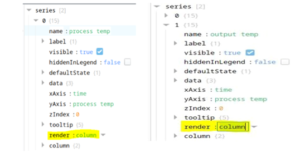Figure 6

### Step: #6

Figure 7.1 should be the result of our efforts.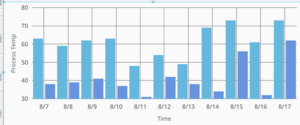Figure 7.1

Now we must select a column and drill down into the column properties and get to: column/appearance/stacked

Then enable the box for stacked.  This needs to be completed in both columns, see figure 7.2.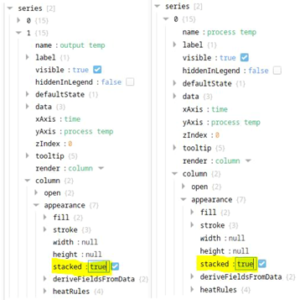Figure 7.2

### Step: #7

When both columns have their stacked properties checked, the chart will display the properly stacked bar chart as shown in figure 8.